# Rectangle

In rectangle with sides, 6 and 3 mark the diagonal. What is the probability that a randomly selected point within the rectangle is closer to the diagonal than to any side of the rectangle?

Result

p =  42.7 %

#### Solution:

$a=6 \ \\ b=3 \ \\ c=\sqrt{ a^2+b^2 }=\sqrt{ 6^2+3^2 } \doteq 3 \ \sqrt{ 5 } \doteq 6.7082 \ \\ \ \\ S_{1}=\dfrac{ a \cdot \ b }{ 2 }=\dfrac{ 6 \cdot \ 3 }{ 2 }=9 \ \\ \ \\ r=\dfrac{ 2 \cdot \ S_{1} }{ a+b+c }=\dfrac{ 2 \cdot \ 9 }{ 6+3+6.7082 } \doteq 1.1459 \ \\ S_{2}=\dfrac{ r \cdot \ c }{ 2 }=\dfrac{ 1.1459 \cdot \ 6.7082 }{ 2 } \doteq 3.8435 \ \\ \ \\ p=100 \cdot \ \dfrac{ S_{2} }{ S_{1} }=100 \cdot \ \dfrac{ 3.8435 }{ 9 } \doteq 42.7051 \doteq 42.7 \%$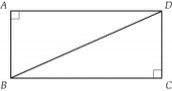Our examples were largely sent or created by pupils and students themselves. Therefore, we would be pleased if you could send us any errors you found, spelling mistakes, or rephasing the example. Thank you!

Leave us a comment of this math problem and its solution (i.e. if it is still somewhat unclear...):Be the first to comment!Tips to related online calculators
Pythagorean theorem is the base for the right triangle calculator.
Would you like to compute count of combinations?

## Next similar math problems:

1. Rectangular land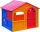On a rectangular land with dimensions of 35 m and 18.5 m is a house with a square floor plan with a side of 14 m. What % of the land is not occupied?
2. Cone roofHow many m2 of roofing is needed to cover a cone-shaped roof with a diameter of 10 m and a height of 4 m? Add an extra 4% to the overlays.
3. The manufacturer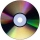The manufacturer found that 3% of the plates produced had a malfunction. Of the compliant, 75% are first and 25% second. What is the probability of producing first and second class plates?
4. The testThe test contains four questions, and there are five different answers to each of them, of which only one is correct, the others are incorrect. What is the probability that a student who does not know the answer to any question will guess the right answer
5. Water percent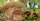A 15 g sample of the substance contains 70% water. After drying, the weight was reduced to 9 g. What percentage of water is now in the sample?
6. What isWhat is the annual percentage increase in the city when the population has tripled in 20 years?
7. Price reductionThe product is sold for 360 CZK and the sales profit is 30%. By what percentage the sales profit will be reduced if I reduce the price of the product by 10%?
8. Double price reduction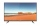The price of TV has been reduced twice. First by 15% and later by another 10% of the reduced price. After this double price reduction, the TV was sold for 8,874 crowns. What was the original price of the TV?
9. All use computerIt is reported that 72% of working women use computers at work. Choose 3 women at random, find the probability that all 3 women use a computer in their jobs.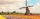How large must the group of people be so that the probability that two people have a birthday on the same day of the year is greater than 90%?
11. ProbabilityHow probable is a randomly selected three-digit number divisible by five or seven?
12. Cllub flag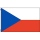The children had the club flag sewn on. The red color covered 2/20 of the total area of the flag, the blue area a fifth, the yellow 19% of the total area. What% is the green area occupied?
13. Christmas holidays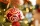How many percent take Christmas holidays lasting 18 days from a school year that lasts 303 days? Round the result to two decimal places
14. Slow saving in banksHow long will it take to save € 9,000 by depositing € 200 at the beginning of each year at 2% interest?
15. The number 72The number 72 increase by 25%. By how much % will you have to reduce the number you created to get the number 72 again?
16. ChestnutsThree divisions of nature protectors participated in the collection of chestnut trees.1. the division harvested 1250 kg, the 2nd division by a fifth more than the 1st division and the 3rd division by a sixth more than the second division. How many tons of
17. ContestantsThe three best contestants are to divide the total prize of CZK 4,200. The second gets 20% more than the third. And the first one gets 200 CZK less than the second and the third together. How much will everyone get?